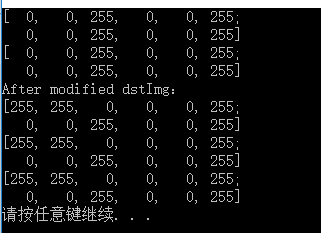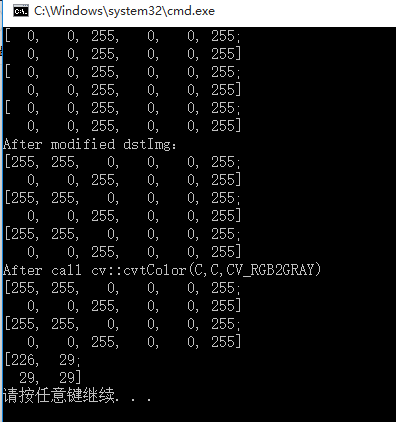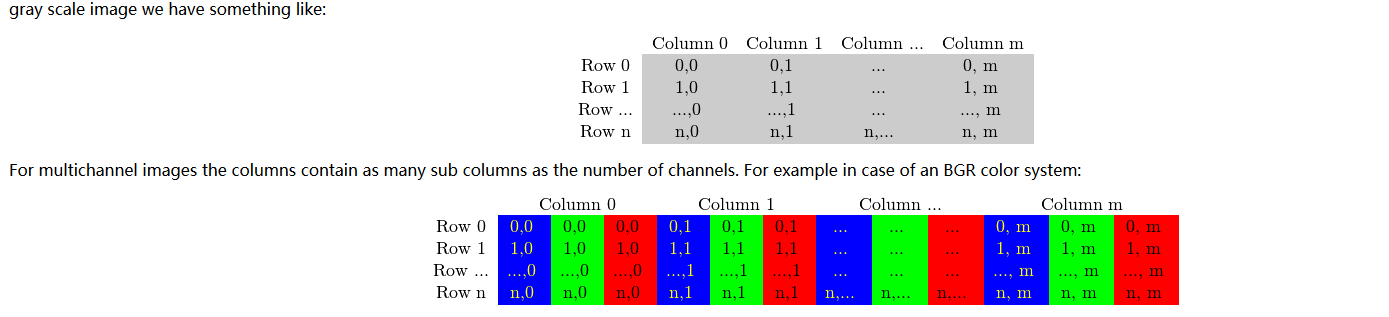# opencv——Mat数据格式及其遍历

在opencv早期的版本中，图像通过一个叫做IplImage的结构（structure）存储在内存中。由于C语言对程序员高度的信任，因此它需要手动地对内存进行管理，比如内存的分配和回收，这在大型程序设计中是比较麻烦的。幸运地是，C++可以很好地帮助程序员管理内存，因此opencv2.0后就引入了C++接口。但是C++也有缺点，比如说目前大部分的嵌入式系统只支持C语言，在这些平台上开发opencv程序的话用C++就不是很好。

int main() {
cv::Mat srcImg(2, 2, CV_8UC3, cv::Scalar(0, 0, 255));
cv::Mat dstImg(srcImg);
cv::Mat C = srcImg;
std::cout << srcImg << std::endl;
std::cout << dstImg << std::endl;
dstImg.ptr<uchar>(0) = 255;
dstImg.ptr<uchar>(0) = 255;
dstImg.ptr<uchar>(0) = 0;
std::cout << "After modified dstImg：" << std::endl;
std::cout << srcImg << std::endl;
std::cout << dstImg << std::endl;
std::cout << C << std::endl;
return 0;
}当然，如果想拷贝矩阵本身也是有办法的，opencv提供了两个方法：clone()和copyTo():

Mat F = A.clone();
Mat G;
A.copyTo(G);

最后总结一下：

1）opencv函数中输出图像的内存是自动分配的；

3）如果想进行深拷贝，即拷贝矩阵本身的数据，可以采用clone()或copyTo()函数。

对1和2的理解可以很重要，这可以解释下面这个程序：

int main() {
std::vector<cv::String> filenames;
cv::glob(path, filenames);
cv::Mat srcImg(2, 2, CV_8UC3, cv::Scalar(0, 0, 255));
cv::Mat dstImg(srcImg);
cv::Mat C = srcImg;
std::cout << srcImg << std::endl;
std::cout << dstImg << std::endl;
std::cout << C << std::endl;
dstImg.ptr<uchar>(0) = 255;
dstImg.ptr<uchar>(0) = 255;
dstImg.ptr<uchar>(0) = 0;
std::cout << "After modified dstImg：" << std::endl;
std::cout << srcImg << std::endl;
std::cout << dstImg << std::endl;
std::cout << C << std::endl;
cv::cvtColor(C, C, CV_RGB2GRAY);
std::cout << "After call cv::cvtColor(C,C,CV_RGB2GRAY)" << std::endl;
std::cout << srcImg << std::endl;
std::cout << dstImg << std::endl;
std::cout << C << std::endl;
return 0;
}

其运行结果为：关于如何创建一个Mat对象，最好的办法就是看mat.hpp，因为实在有太多了...，这里在介绍一下opencv里面的一下data

type，比如说CV_8UC3,CV_32FC3,CV_32F是什么意思：

CV_[the number of bits per item][signed or unsigned][Type prefix]C[The channel number]

最后是一个大头部分：介绍如何遍历cv::Mat。

Q1:图像在Mat中是如何存储的呢？

A1:Mat& ScanImageAndReduceC(Mat& I, const uchar* const table)
{
// accept only char type matrices
CV_Assert(I.depth() != sizeof(uchar));

int channels = I.channels();

int nRows = I.rows * channels;
int nCols = I.cols;

if (I.isContinuous())
{
nCols *= nRows;
nRows = 1;
}

int i,j;
uchar* p;
for( i = 0; i < nRows; ++i)
{
p = I.ptr<uchar>(i);
for ( j = 0; j < nCols; ++j)
{
p[j] = table[p[j]];
}
}
return I;
}
MatObject.ptr<type>(rowIndex)[columIndex] = a number


04-0607-08
11-101万+
03-301万+
01-062万+
05-051554
12-011863
04-164万+
01-07711
05-021万+
03-299153
11-071万+
08-023349
07-162588
03-111298
04-1310万+
11-191838